Edward Nightingale is a master woodworker and woodcarver with over 30 years of experience using the tools of the trade, measure and geometry, as well he has been a performing musician for over 30 years. Applying his understanding of geometry, measure, mathematics and music, he has discovered designed in the geometry and layout of the pyramid complex at Giza, by the original architects, high science and mathematics. One of the mysteries solved in The Giza Template is the root of the measuring system, how the original numbers are derived and their relationship to the speed of light. See Edward’s website at: http://www.thegizatemplate.com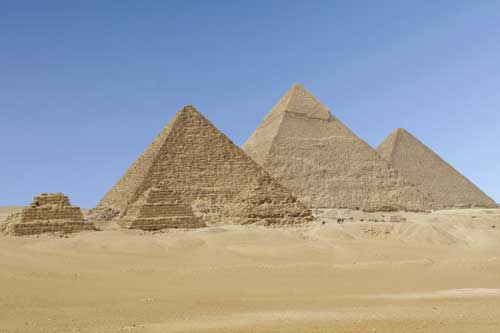In 1997 I traveled to Egypt with John Anthony West with an idea on the design and layout of the pyramid complex at Giza. I hypothesized that Giza was designed as a whole complex, with each structure playing its part and information would be encoded within the geometry of the design, which could then be translated into mathematics. The fact that there are no hieroglyphs or inscriptions at the site is logical, hieroglyphs and inscriptions would detract from the geometrical information encoded. After many years of studying the Giza Plateau I have complied evidence to show that there is indeed information encoded at Giza and I will present this new information in my work, The Giza Template, Key of 432. This article will cover the geometry and the Phi, Pi, Fibonacci relationship.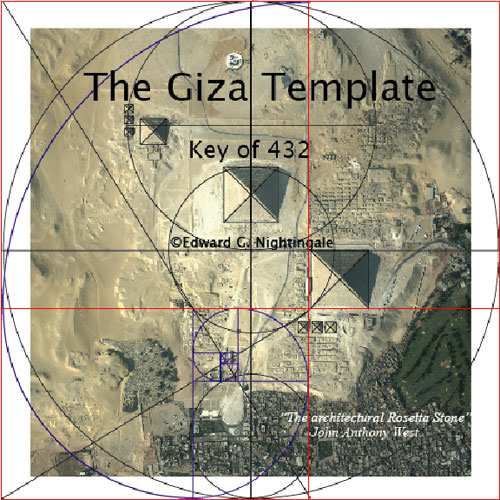The E-book

To back engineer the design of the Giza Plateau, we do so with the following understanding as best explained by John Neal. The original architects were to “design a model of the world durable enough to be interpreted without instruction five or more millennia into the future”. “The tools used by the original creator are regarded as a straightedge and a compass and he who would attempt the task would be limited by the same constraints. The solution to the reconciliation of arcs and lines must therefore be governed by proportion”. “These ideal expressions may be used for convenient approximations that are easily committed to memory, the tools of metrology, or number system are then available to correct the figures to any degree of accuracy”. Working with a compass and straight edge then verifying with Auto Cad, I re-drew the Giza Plateau and have uncovered the key to understanding the message encoded.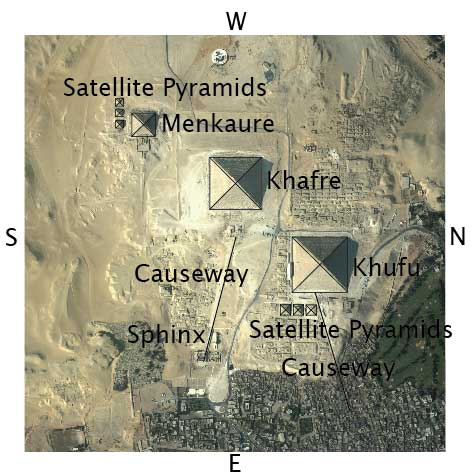To familiarize your self with the Giza Complex, it is viewed looking down, in its architectural plan view. The three main pyramids are, Menkaure, the smallest of the three in the in the upper left, the Pyramid of Khafre, the second smallest in the center, and the Great Pyramid of Khufu in the lower right. There are three small Satellite Pyramids associated with Khufu, as well three small Satellite Pyramids located next to the Pyramid of Menkaure. There are two causeways, the Sphinx, and Khufu causeways with the Great Sphinx located near the bottom of the image.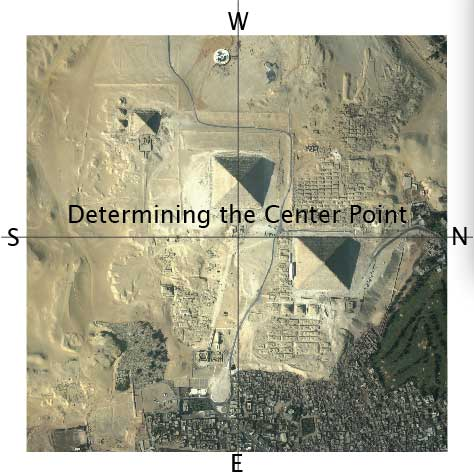To begin we’ll use a Quickbird Satellite © photo of the Giza Plateau that includes but is not limited to 9 pyramids and The Great Sphinx. Notice the orientation, West is at the top, going clockwise, North to the right, East toward the bottom and South to the left. To determine the center point we draw a line from South to North along the Western face of The Pyramid of Khufu, the great pyramid. This line also intersects the point at which the angled causeway leading to the Sphinx begins. Drawing a line from West to East bisecting the center of The Pyramid of Khafre we once again intersect the angled causeway leading to the Sphinx.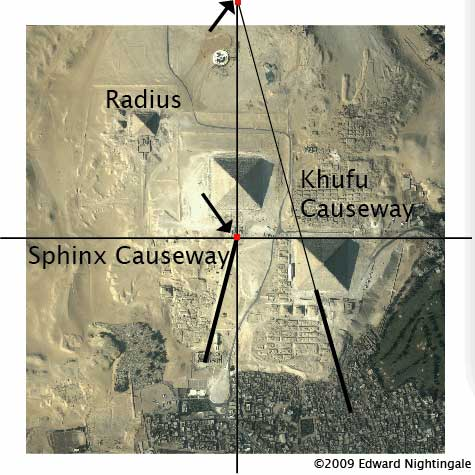These three intersecting points seem to suggest the axis of a circle, with the angled Sphinx causeway pointing to circles center. Based on the same logic the angled causeway leading from the Eastern face of The Pyramid of Khufu is extended to the point west where it intersects the East West centerline. These two points are used to determine the radius of the circle.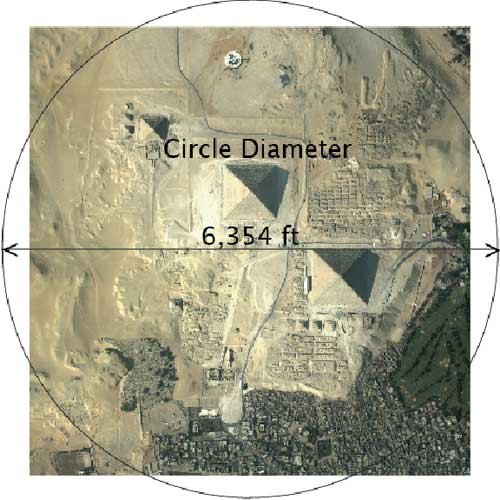The radius of the circle creates a diameter of 6,354 ft. in full scale using Auto Cad.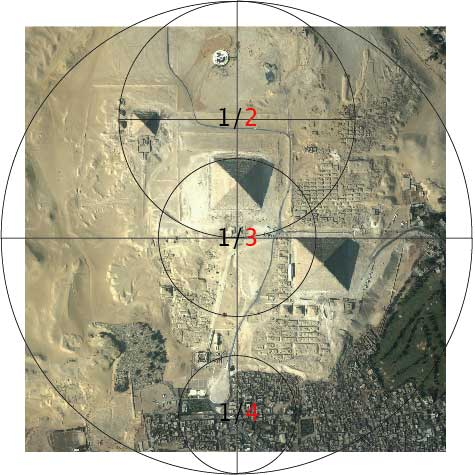If our hypothesis is correct and our circle is relevant, proportions of the main circle should coincide with relative structures on the plateau. As we can see, circles with proportions of ¼, ? and ½ do coincide. The ¼ diameter circle aligns with the Sphinx, the ? diameter aligns with the Pyramid of Khafre and the Pyramid of Khufu, and the ½ diameter circle aligns with the three satellite pyramids of Menkaure. These proportionate circles give us a clue to the next step in deciphering the encoded mathematics.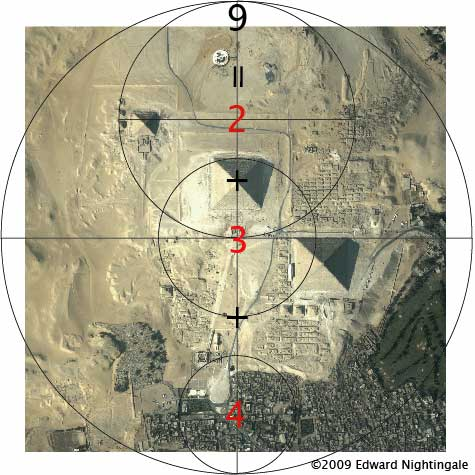Our last step was to divide the circle using proportion, if we add the three numbers that are given in the last step, 4,3 and 2 we have the sum of 9 (4+3+2=9). To see if the number 9 has any relevance to the circle and structure alignments we have several options. We can use the number 9 to divide the circles circumference, its radius, or its diameter. A note here is that this specific geometry also allows the circumference of the circle to be divided by 9 and is covered in depth in The Giza Template.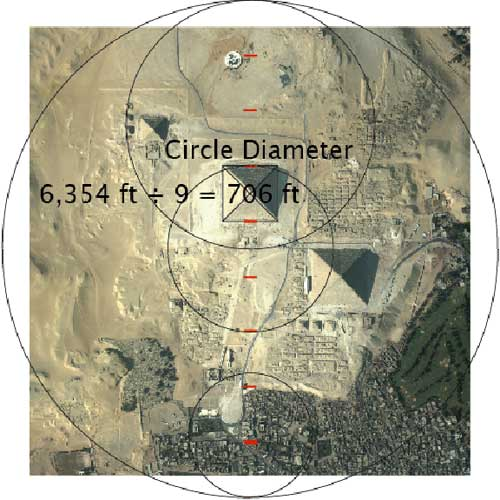We have determined the diameter of the circle to be 6,354 ft. using Auto Cad; if we divide 6,354 ft. by 9 we get 706 ft. It becomes obvious that dividing the main circles diameter by 9 is relevant to the structures; the base length of the Pyramid of Khafre is 706 ft. and is located exactly in the 6th position up from the bottom of the circle.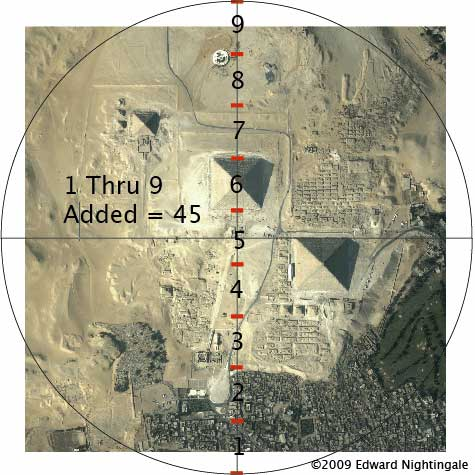Following the same train of thought, adding the numbers derived from the previous step, we add 1 through 9 together and have the sum of 45. 1+2+3+4+5+6+7+8+9=45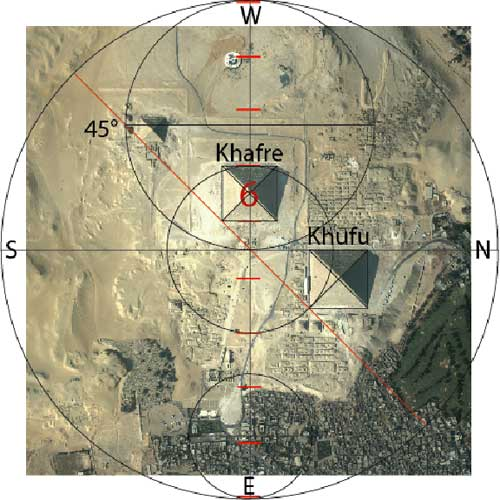So what does the number 45 have to do with a circle? 45 can be seen as 45° degrees of angle within the circle. If we draw a 45° degree angled line from the South West corner to the North East corner of the main circle we can see that the line intersects the South Eastern corners of both the Pyramid of Khafre and the pyramid of Khufu.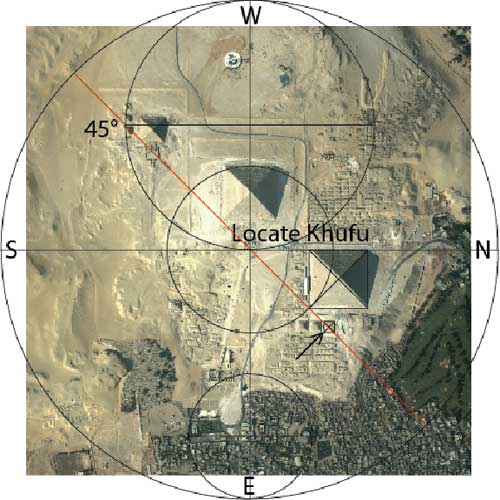Using the intersection of the South East corner of Khufu and drawing a line vertically to the horizontal center line we determine the base length*of the pyramid. One note at this point, *the full-scale measurement is not the exact base length of the Pyramid of Khufu which is 756 ft. but is completely relevant when the relationship of the elevation of the pyramid base to the elevation of the axis of the circle are taken into account. This will be addressed at a later point and is very important and elegant in its design. We also note that the 45°degree line intersects the center of the northern most satellite pyramid of Khufu.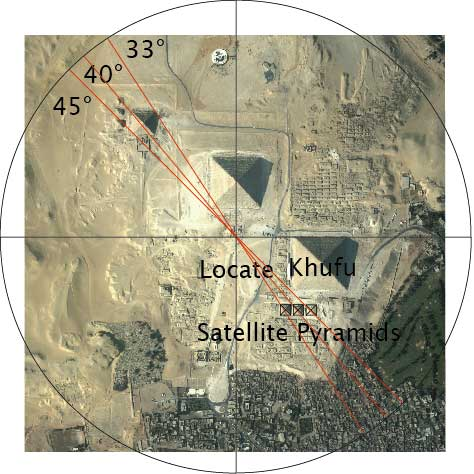If we draw lines through the axis of the circle and through the centers of each of the other two satellite pyramids of Khufu we find the first line angle was 45° degrees, the second line angle is 40° degrees and the third line angle is 33° degrees. If we ask what these three numbers have to do with a circle, the answer is it divides the main circles 360-degree circumference by 8, equaling 45° degrees, dividing by 9 equaling 40° degrees and dividing by 11 equaling 32.727272° degrees. A note here is the numbers 11, 32 and 33 will be covered in depth at a later point in the Giza Template and are very relevant.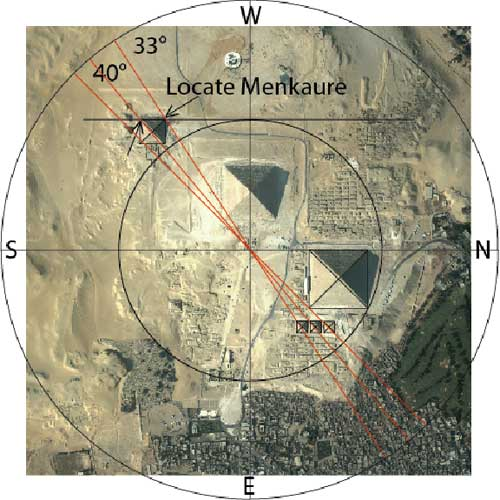As we can see the 40°degree and the 33°degree angled lines intersect the South West and North West corners of the Pyramid of Menkaure. By drawing a circle with its axis at the center of the main circle and its radius equal to the distance from the main circles axis the North East corner of the Pyramid of Khufu, the radius of this circle determines the distance of the Western face of the Pyarmid of Menkaure from the horizontal South to North centerline. This particular circle diameter will also be relevant at a later point when dealing with the Phi Ratio and is explained in depth in the Giza Template.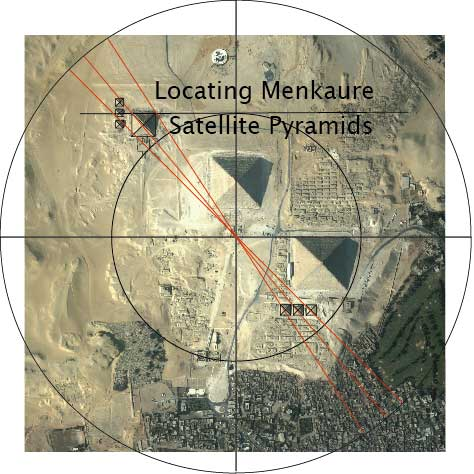Locating the satellite pyramids of the Pyramid of Menkaure will be addressed in The Giza Template and is very exact in their placement and verifies other steps in decoding the mathematical message.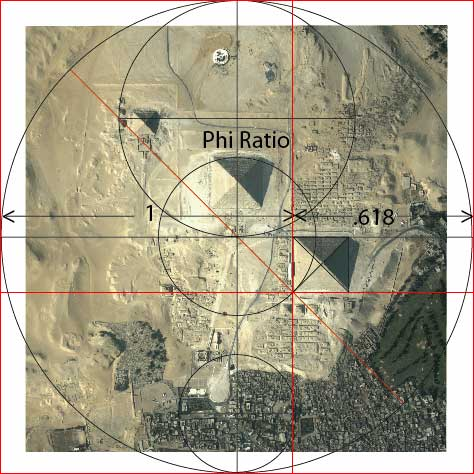Up to this point we have been working with the main circle, its divisions and proportions, we determined the main circle to be 6,354 ft. in diameter. Now focusing on the Pyramid of Khufu, if we draw a horizontal line intersecting the South East corner of the Pyramid of Khufu, the 45° degree angled line and the intersection of the 1/3 diameter circle. Then drawing a vertical line at the same intersection, we divide a square with a side length of 6,354 ft. the same as the main circle diameter, 6,354 ft. into its Phi Ratio, 1 to .618. So we can state, simply, the South Eastern corner of Khufu divides the square of 6,354 ft. into its Phi Ratio point.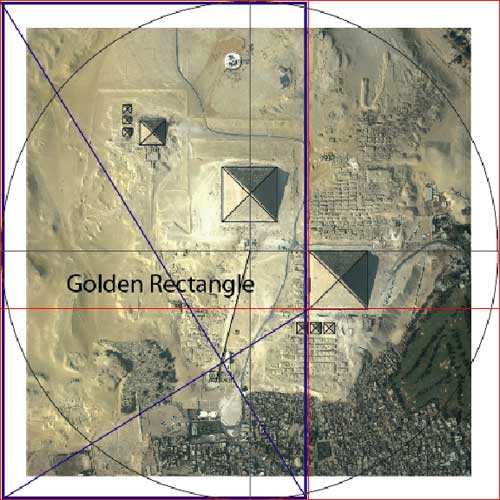We have addressed the main features (pyramids) in the Giza Complex with the exception of the Great Sphinx. If we draw 2 diagonal lines, one from the upper left corner of the square to the bottom right corner of its Phi division, and another line diagonal from the bottom left corner of the square to the South East corner of the Pyramid of Khufu we can see the these two diagonal lines intersect with the angled causeway to the Sphinx which was our first indicator to the axis of the circle. These diagonal lines are drawn within a Golden Rectangle created by dividing the square at its Phi ratio point. This Golden Rectangle is the then used to create a Fibonacci Spiral that demonstrates the relationship of the Phi Ratio to the Fibonacci Series. The Fibonacci Series is a method of adding two like numbers together, finding its sum, then adding the previous number to it to get the next sum, for example; 1+1=2, next add 1+2=3, next 2+3=5, next 3+5=8, 5+8=13, 8+13= 21 and so on. For this example the Fibonacci Series is 1, 1, 2, 3, 5, 8, 13, 21. This sequence can be done starting with any 2 like numbers and will be discussed in detail in the Giza Template.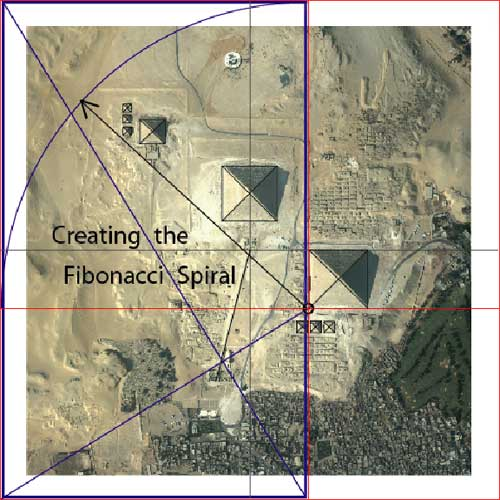The next 2 illustrations will show the creation of the Fibonacci Spiral and its relationship to the location of the Great Sphinx.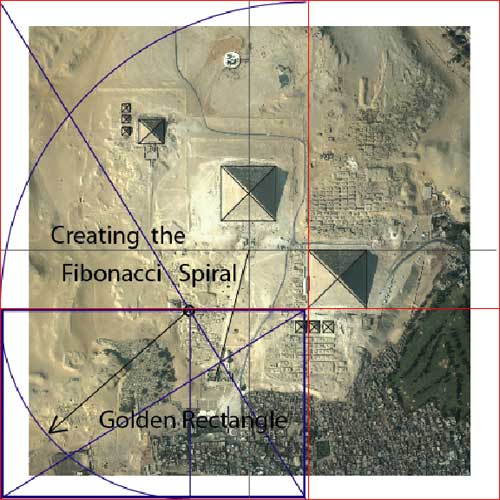The next step or axis is determined by the intersection of horizontal Phi division line and the diagonal line within the Golden Rectangle. At this intersection, the axis, we draw a radius equal to the distance to the edge of the square. This also creates another smaller Golden Rectangle in the horizontal position. The axis of the next radius is located at the Phi division line. This process is repeated and will scale down, or up in its size to infinity.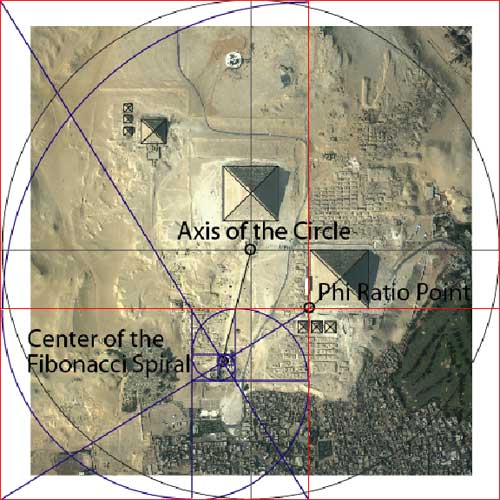It is important to note here how the three center points, the axis of the circle, the Phi Ratio point of the Square, and the Golden Rectangle placing the Fibonacci Spiral center point all work together. Nature uses the Phi Ratio and the Fibonacci sequence to construct itself in this scaling manner. Some examples of the Fibonacci sequence are found in such things as nautilus shells, pinecones, sunflowers, the human ear and DNA. The Phi Ratio can be seen in the proportions of the human body and one example is the forearm. The distance from your elbow to your wrist is equal to 1 unit. Then, the distance from your wrist to your fingertip is equal to .618 of a unit, the Phi Ratio of 1 to .618. This is an easy way to remember the Phi Ratio and happens to be the hieroglyph for the Egyptian Cubit a unit of measure built within the Pyramid of Khufu and is very well documented by scholars. A note here, the origin of the Cubit and the measuring system are covered in depth in The Giza Template.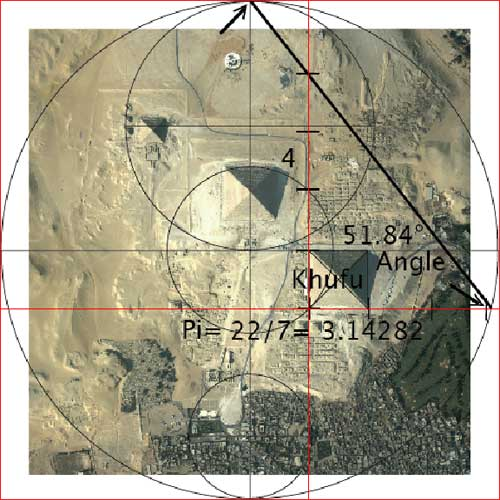There is another wonderful relationship here, and that is the main circles relationship to the Phi Ratio division of the square. We draw an angled line from the top of the circle at its centerline to the point at which the circle and horizontal Phi division line intersect. The angle created here is 51.84° degrees, the angle of the Pyramid of Khufu. This angle can be expressed in a pyramid with its ½ base length being 3.14282units and its height being 4 units. The fraction of 22/7 was used by the Egyptians to express Pi and its decimal equivalent is 3.14282. This has been a brief introduction to the geometry encoded within the Giza Complex and its mathematical message. We’ve seen the main circle diameter divided by nine, why into ninths? The decimal equivalent to 1/9 is .111111 to infinity, and 2/9 is equal to .222222 to infinity, 3/9 equals .333333 to infinity and so on. These numbers are the ultimate numbers used to scale, or to grow in size without changing shape. We have seen that the Phi ratio also divides and scales the square up or down in size infinitely. The Fibonacci Series, its “occult” or hidden center scales the spiral up or down to infinitely. These perfect scaling numbers and the associated geometry are at the core of the information encoded at Giza, with many more levels and facets hidden within the template. In The Giza Template Key of 432 I present the information I have discovered to date, including but not limited to: the origin of measure, its relationship to the music scale, its connection with the speed of light and evidence of the ancient high science encoded. A clear chain of custody of this ancient knowledge is shown using the precise geometry and numbers used in symbolism, sacred numbers and heraldry. This and much more are revealed in “The Giza Template, Key of 432”. For more information please visit my site: http://www.thegizatemplate.com

Edward Nightingale is an independent researcher, author and speaker who as a Master Woodworker with over 33 years of drawing, measuring and building experience has spent the past 17 years utilizing these skill sets to reverse engineer the Giza Complex.

Edward travelled to Egypt in 1997 in search of evidence for his theory on the architectural design of the Giza pyramids and the Sphinx, the results of his efforts are published in his new book, The Giza Template: Temple Graal, Earth Measure. The book documents the step by step design that the architects of Giza used to encode highly scientific data into geometric and mathematical information to be preserved and passed on to future generations.

Edward’s claims are backed with precise geometry, mathematics, satellite imaging, astronomical charting and cosmology that can be reproduced using the scientific method.

Edward is working on a sequel, Heavens Measure to follow his book Earth Measure.

Website: www.thegizatemplate.com